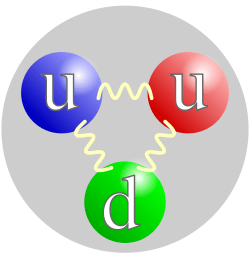## From Fermilab- “Frontier Science Result: DZero Which way did it go?”Fermilab is an enduring source of strength for the US contribution to scientific research world wide.

Thursday, Aug. 28, 2014
Leo Bellantoni

In the DZero detector., the direction in which a particle travels — the direction of its momentum — is often easier to measure precisely than its energy or, equivalently, its amount of momentum. This is the key idea behind a recent result.

Put a flat piece of paper on your desktop. This is what particle physicists call the transverse plane. Now take a pencil and try to balance it on its point on the paper. You don’t have to succeed — just get the idea of something going perpendicularly into the paper. This depicts the proton going into the collision. The antiproton is traveling in the opposite direction, also perpendicular to the paper (excuse me: also perpendicular to the transverse plane). If the two are aligned and strike each other, then there is a collision point in the transverse plane. Pick the pencil up; there might be a little mark left from the pencil tip to show you where the collision happened.The quark structure of the proton. (The color assignment of individual quarks is not important, only that all three colors are present.)The quark structure of the antiproton.

Now let us draw some arrows coming out from that little mark. These are the directions of the particles coming out of the collision. By drawing them on the paper, you draw only two dimensions of the motion — a particle might come out with a motion that is partly upwards and to the left, but on the paper you can only draw it to the left. This is what particle physicists call projecting onto the transverse plane.In the above figure you see a pair of possibilities for the projection onto the transverse plane of two particles from a collision. These two particles are the decay products of a very heavy particle created in the collision. The two particles on the left came from some heavy particle that was clearly headed south according to the compass. The two particles on the right came from a heavy particle that was clearly going nowhere.

The method of the recent DZero result is a bit more complicated than this, but you have enough to get the basic idea. When a proton and an antiproton collide and produce a Z boson, the Z boson might decay into a pair of muons. The direction of the muons tells us how the Z was moving in the transverse plane. What scientists actually do is take the angle that you see in the figure, which is called the acoplanarity, and apply a certain correction to it to obtain an angle that theorists can predict and experimentalists can measure. More to the point, this angle, called Φ* and invented in part by DZero collaborators, is experimentally a more precise measure of the motion of the Z (as projected onto the transverse plane) than that obtained by measuring just the momentum of the muons in the transverse plane directly.

The theoretical prediction relies on being able to calculate the effects of the strong nuclear force accurately, and this is a notoriously difficult thing to do. So in this case, comparing the data to the prediction is more a test of our ability to apply the theory than a test of the theory itself.

DZero finds that the data and the theoretical predictions are in good but not perfect agreement. At Φ* near 30 degrees, the DZero measurement is higher than the prediction, but the prediction is not very definite. Now it is the theorists’ turn to try to improve our ability to apply the theory.